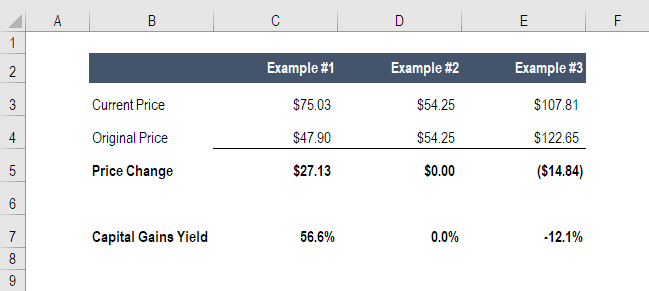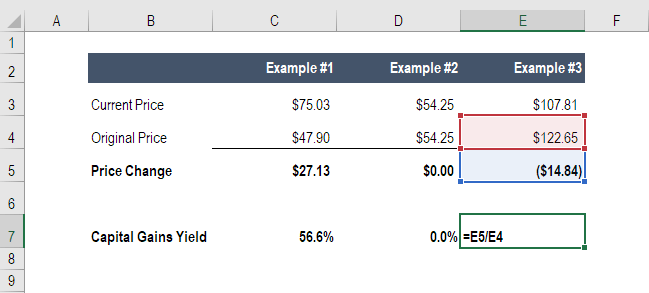# Capital Gains Yield

Capital gain expressed as a %

## What is Capital Gains Yield (CGY)?

Capital gains yield (CGY) is the price appreciation on an investment or a Security expressed as a percentage.### Capital Gains Yield Formula

CGY = (current price – original price) / original price x 100

Capital Gain is the component of total return on an investment, which occurs as a result of a rise in the market price of the security.

Below is a screenshot of the formula used to calculate CGY (the same numbers as the example above).### Calculating Capital Gains Yield

Consider the following example. John buys a share of company XYZ at market price of \$100. Over the course of one year, the market price of a share of company XYZ appreciates to \$150. At the end of the year, company XYZ issues a dividend of \$5 per share to its investors.

The Capital Gain Yield for the above investment is (150-100)/100 = 50%.

Also note that:

The Dividend Gain Yield for the above investment is 5/100 = 5%.

The total return from the investment is therefore 55%.

### Interpreting Capital Gain Yield

It is difficult to say much about an investment from its Capital Gain Yield alone. We have seen how it is possible for the Total Gain from an investment to be larger than the Capital Gain. However, we have not commented on the size of this difference.

Let us analyze an extension of the previous example. Unlike John who invested in company XYZ, Mark invested in company ABC, which had a market price of \$100 per share at the start of the year. At the end of the year, company ABC has a market price of \$105 per share. In addition, company ABC issues a dividend of \$50 per share.

The Capital Gains Yield for Mark’s investment is (105-100)/100 = 5%, which is much less than the 50% that John receives.

However, Mark’s dividend gain yield is 50/100 = 50%, which is greater than what John receives.

Which investment would be considered superior?

Both John’s investment into XYZ and Mark’s investment into ABC give a total gain of 55%. Thus in isolation, it is difficult to interpret much about an investment from its Capital Gain Yield alone. In fact, it is possible for an investment to give a positive total return (total gain) despite posting a capital loss.

Consider the case where the price of company ABC falls from \$100 to \$95, but ABC gives a dividend of \$60 per share. The total gain would remain 55% (5% capital loss and 60% dividend gain).

In practice, every dollar that is paid out as a dividend is a dollar that the company cannot reinvest back into its business. Therefore company XYZ will be seen as a company with high growth potential because profits of XYZ are redirected back into the company’s growth and not distributed to shareholders, whereas company ABC will be seen as a company with low growth potential because its profits are distributed to shareholders and not used for company growth.

See our free guide to becoming a world class financial analyst.

### Practical Applications of Capital Gain Yield (CGY)

Because the calculation of Capital Gain Yield only involves the market price of a security over time, it can be used to analyze the degree of fluctuation in the market price of a security.

Previously, we looked at two investments: John’s investment into XYZ and Mark’s investment into ABC. Both of these securities, XYZ and ABC, have generated income for John and Mark, respectively. Thus John and Mark both must pay tax on this income.

A change in the market value of a security is not relevant for tax purposes until it is realized as a capital gain (or loss) by sale or exchange.

If a security purchased for \$100 appreciates to a value of \$150 in a year, no tax is due on the unrealized capital gain. But if it is sold for \$170 two years after purchase, the difference of \$70 must be declared as capital gains realized at the time of sale, and tax must be paid at the rate applicable to it.

For simplicity, let us assume that both of the investments considered were undertaken for the period of one year.

If capital income is taxed at 10% and dividend income at 15%, which investor between John or Mark is better off?

 John’s Investment in XYZ Mark’s Investment in ABC Capital Gain = \$50 Capital Gain = \$5 Capital Gains Tax = (10/100)*50 = \$5 Capital Gains Tax = (10/100)*5 = \$0.50 Dividend Gain = \$5 Dividend Gain = \$50 Dividend Gain Tax = (15/100)*5= \$0.75 Dividend Gain Tax = (15/100)*50 = \$7.50 Total Gain = \$49.25 Total Gain = \$47

We can see that when there exists a capital gains tax of 10% and a dividend gain tax of 15%, then John’s investment is superior to Mark’s. Generally, dividend gain is considered ordinary income and thus, is usually taxed at a progressive rate.

• Capital Asset Pricing Model
• Dividend Income
• Retained Earnings
• Financial modeling guide

### Financial Analyst Training

Get world-class financial training with CFI’s online certified financial analyst training program!

Gain the confidence you need to move up the ladder in a high powered corporate finance career path.

Learn financial modeling and valuation in Excel the easy way, with step-by-step training.